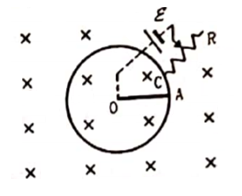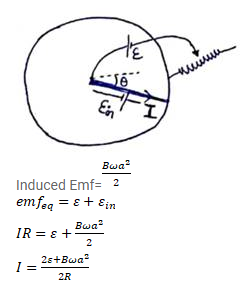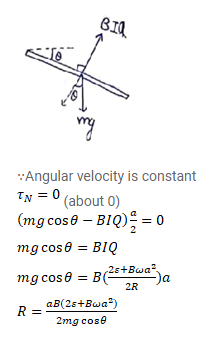# Figure shows a situation similar to the previous problem.

Question:

Figure shows a situation similar to the previous problem. All parameters are the same except that a battery of emf $\varepsilon$ and a variable resistance $\mathrm{R}$ are connected between $\mathrm{O}$ and $\mathrm{C}$. he connecting wires have zero resistance. No external force is applied on the rod (except gravity, forces by the magnetic field and by the pivot). In what way should the resistance $R$ be changed so that the rod may rotate with uniform angular velocity in the clockwise direction? Express your answer in terms of the given quantities and the angle $\theta$ made by the rod OA with the horizontal.Solution: